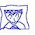Thursday, March 26, 2009

Math Poems

I was in a mood one day, and this was my response to a math question...
(Do you know any math poems?)

Imaginary Numbers Do the Trick
by Sue VanHattum
(written on December 15, 2008)

In an email group of 4,000 homeschoolers,
a member wrote:
My son asks, “The square root of 1 is 1,
So what's the square root of -1 ?”
This was my reply to her…

What we call the real numbers
is everything on a number line,
positive, negative, zero.

If you're thinking about those real numbers,
on that number line,
none of the negative numbers can have a square root,
because anything times itself will come up positive
(or zero).

But, once upon a time (for real),
mathematicians dueled
by giving each other lists of thirty hard problems.
The winner got recognition
and perhaps a job.

All this dueling led to
a solution for cubic equations:
these mathematicians
created a formula
that would find the numbers
that would solve a thing
like 2x3-3x2+4x-5 = 0.

But that formula was a problem!
It came up with square roots of negative numbers,
which drove the mathematicians wild.
No, no, no. There is no such thing!
Well, maybe there could be…
and if there is,
what would it look like?

With a wave of the magic wand of imagination,
These mathematicians
which later got the name i.
(Imagine it written in fancy script.)
i is the square root of -1,
so i squared must equal -1.
i is the first step in creating …
the imaginary numbers.

Picture, if you will, a new number line
of imaginaries
crossing the line of real numbers at 0,
with the real number line horizontal,
and this new one vertical.
(It looks just like x and y axes,
but now it's all one number system,
a bit more complex.)

i sits one step above zero.
Another step up this imaginary number line,
we see 2i,
2i is the square root of -4.
(It is?!
Why yes, 2i times 2i equals 4 times i squared,
and i squared equals -1,
so we get -4.
Cool, huh?)
And on it goes.

Now all of this wouldn't really solve much
if there were no square root of i,
and that seems too weird to think about.
But, once you study trigonometry
(how'd that get in here?!),
the solution to that little problem
is actually quite elegant.

1.I love the perspective! It's cool to see a math person who sees the poetry in numbers.

2.3.